### Adversarial Attacks against Deep Learning Models

Research Question: Is Deep Learning secure for Robots?• Background

Han Wu    @wuhanstudio

Ph.D. Student at the University of Exeter, in the U.K.

## Background

Is Deep Learning secure for Robots?

Intelligent Robots: Deep Learning in Robotics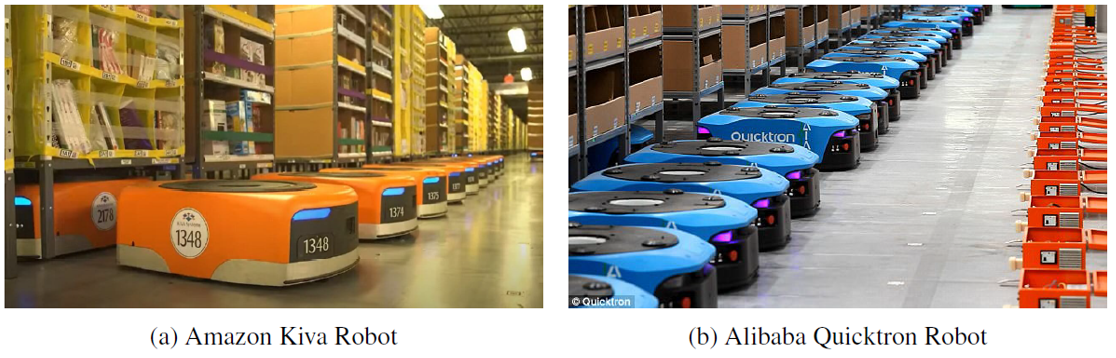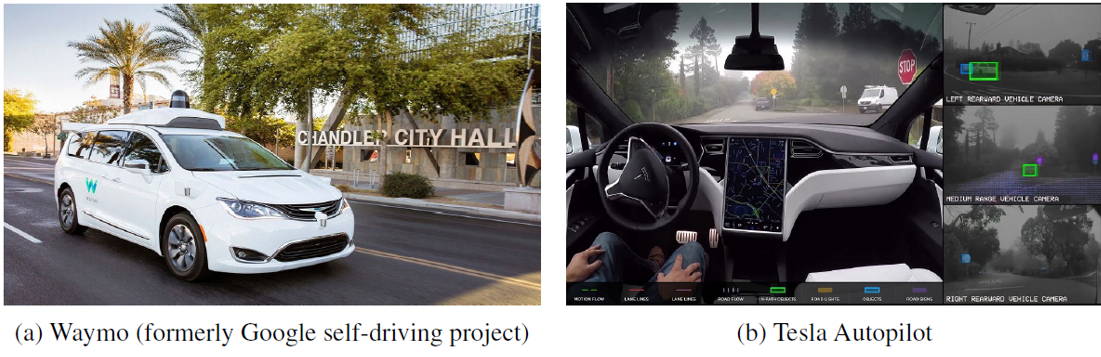Deep Learning for Autonomous Driving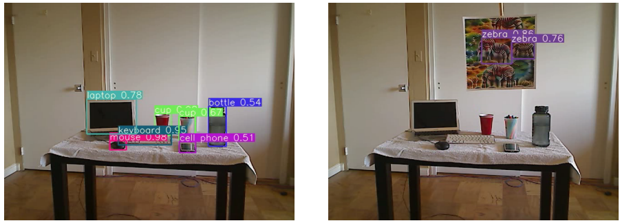Adversarial attacks against object detection

Deep Learning Models are differentiable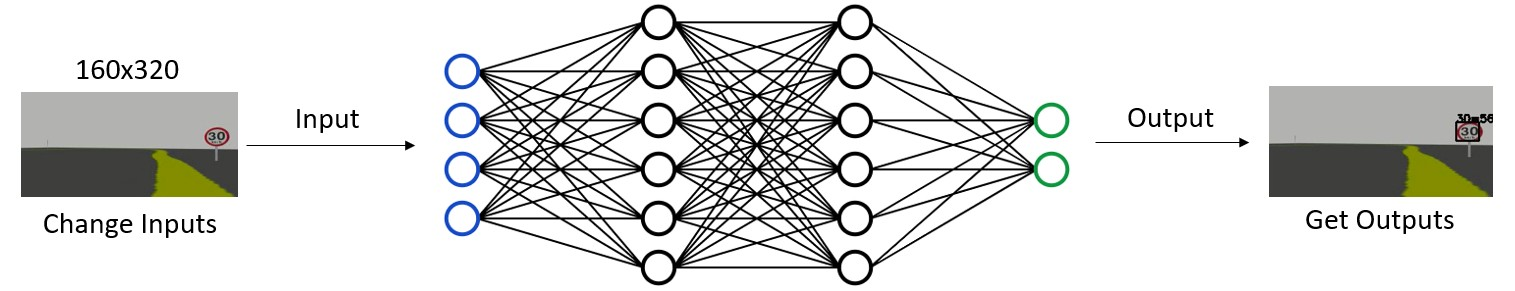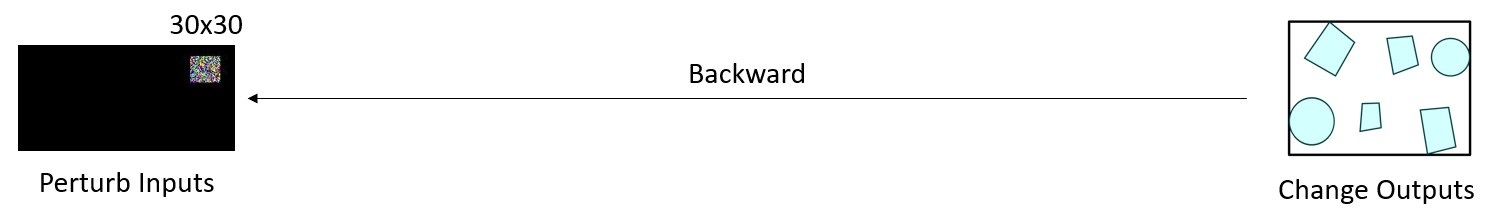Deep Learning Models are differentiable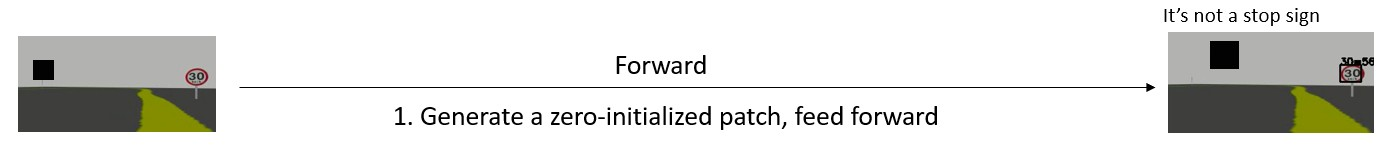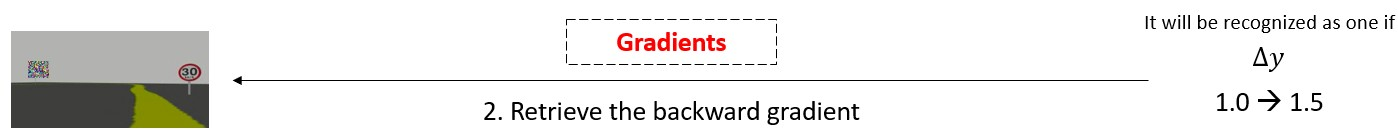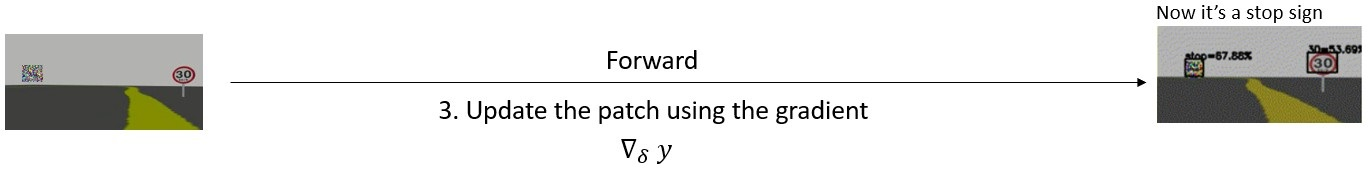Deep Learning Models are differentiable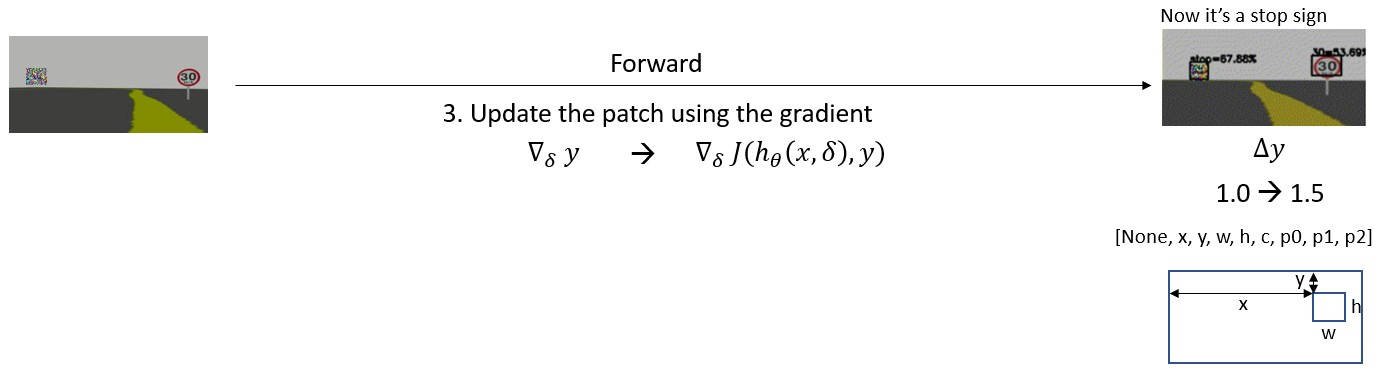One Targeted Attack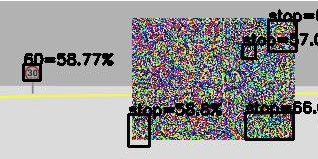Multi Targeted Attack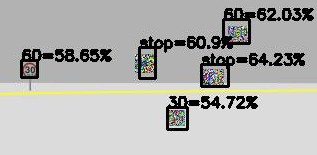Multi Untargeted Attack

## Adversarial Detection: One Targeted Attack

$$J_1(x, \delta, y_h) = max(\ \sigma(c) * \sigma(p_0) \ )$$

                        
# One Targeted Attack
loss = K.max(K.sigmoid(K.reshape(out, (-1, 8))[:, 4]) * K.sigmoid(K.reshape(out, (-1, 8))[:, 5]))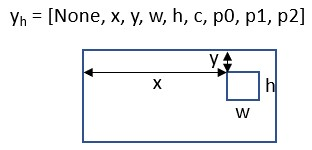## Adversarial Detection: Multi Targeted Attack

$$J_1(x, \delta, y_h) = max(\ \sigma(c) * \sigma(p_0) \ )$$

$$J_2(x, \delta, y_h) = \sigma(c) * \sigma(p_0)$$

                        
# Multi Targeted Attack
loss = K.sigmoid(K.reshape(out, (-1, 8))[:, 4]) * K.sigmoid(K.reshape(out, (-1, 8))[:, 5])## Adversarial Detection: Multi Untargeted Attack

$$J_1(x, \delta, y_h) = max(\ \sigma(c) * \sigma(p_0) \ )$$

$$J_2(x, \delta, y_h) = \sigma(c) * \sigma(p_0)$$

$$J_3(x, \delta, y_h) = \sigma(c) * \sum\sigma(p_i)$$

                        
# Multi Untargeted Attack
loss = K.sigmoid(K.reshape(out, (-1, 8))[:, 4]) * K.sum(K.sigmoid(K.reshape(out, (-1, 8))[:, 5:]))